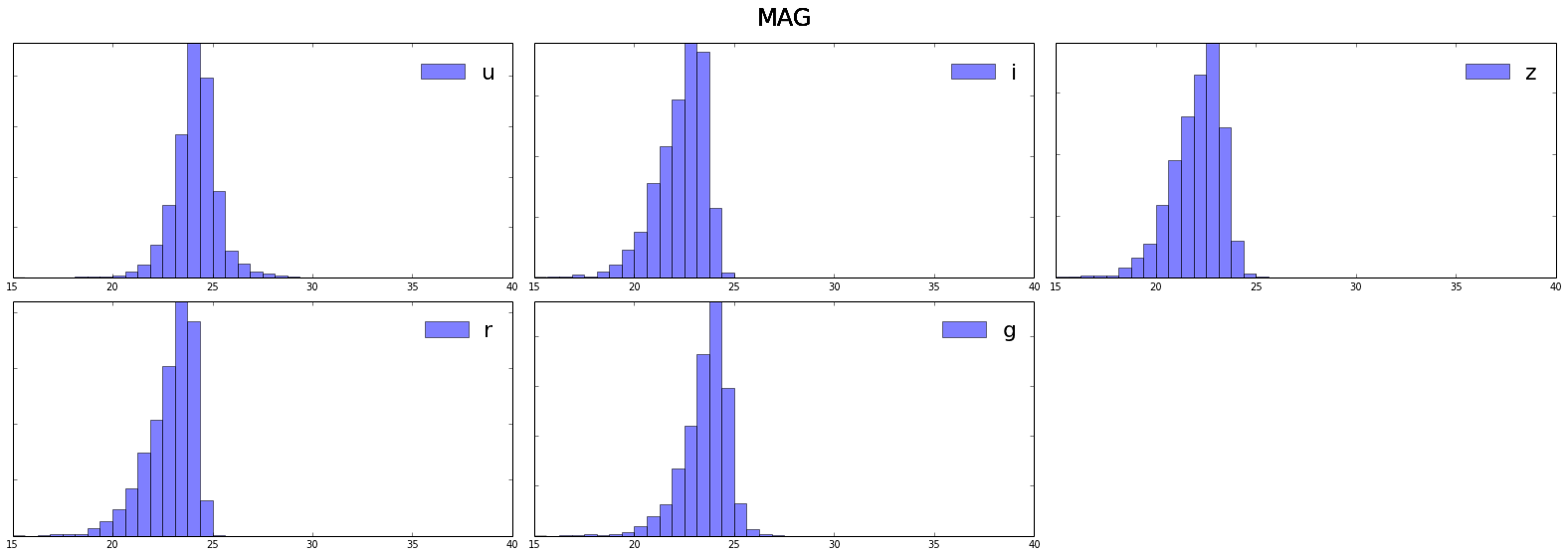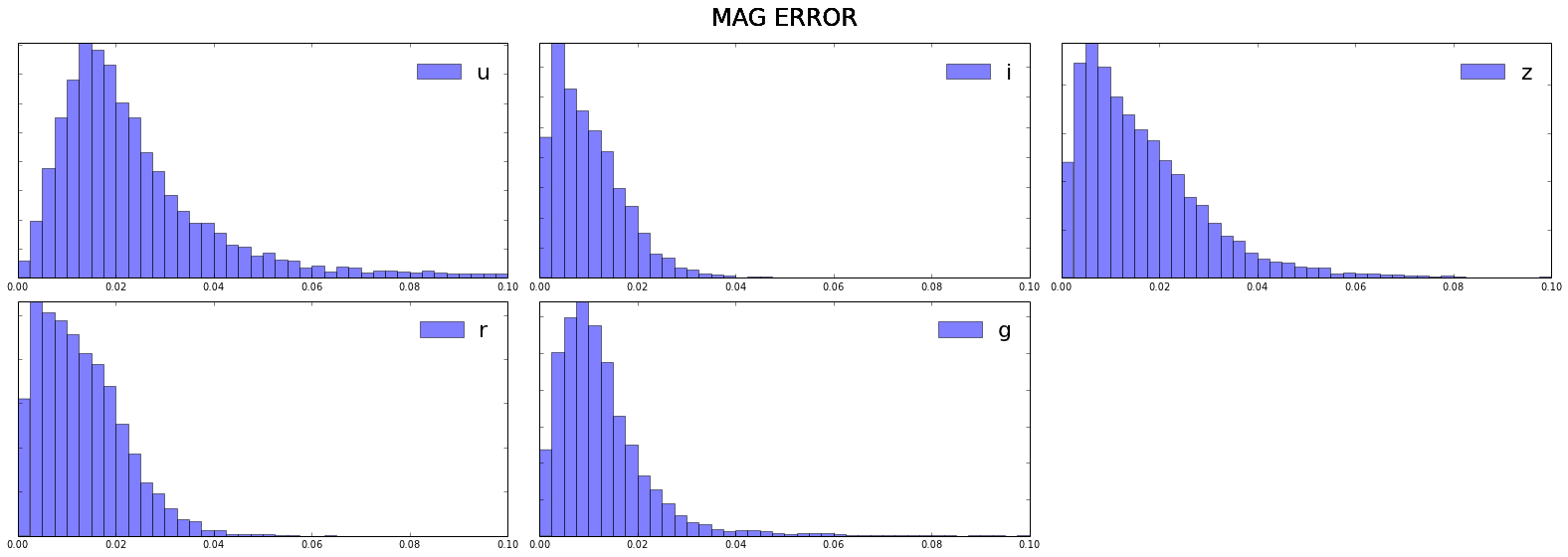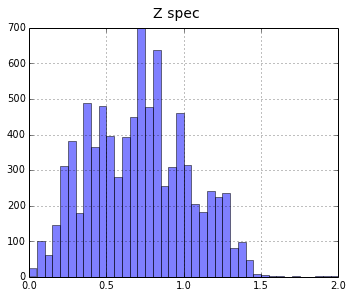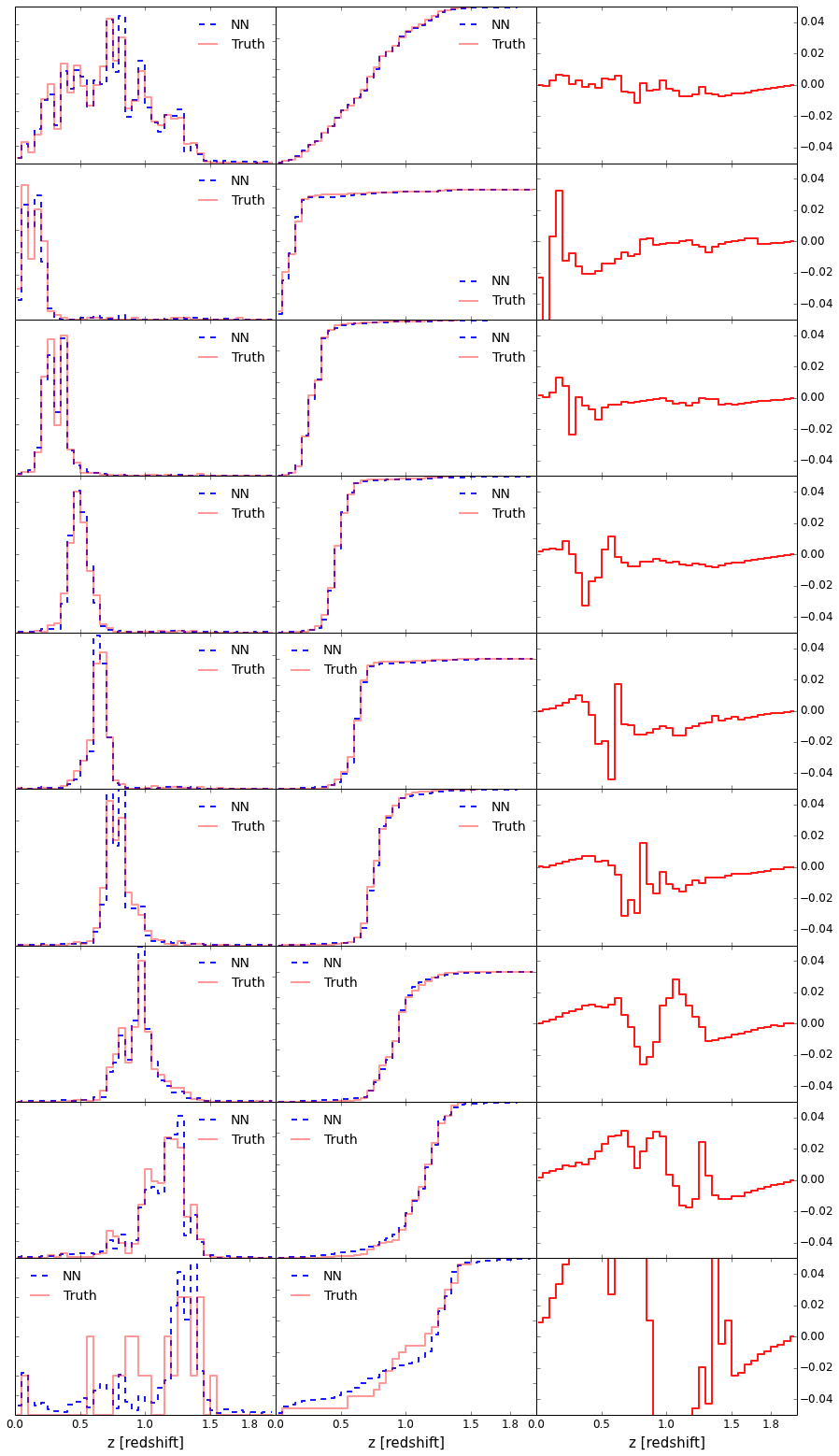# Classification Neural Network for Photo-Z's using SkyNet with the T-800 notebook¶

Author : Christopher Bonnett
contact : c.bonnett [at] gmail.com

Skynet is a fictional, self-aware artificial intelligence system which features centrally in the Terminator franchise and serves as the franchise's main antagonist. Scarcely depicted visually in any of the Terminator media, Skynet's operations are almost exclusively performed by war-machines, cyborgs (usually a Terminator), and other computer systems, with a continuing goal being the extinction of the human race.

Welcome to the notebook accompanying this paper
It will show you how to get Photo-Z's with SkyNet as a regression network and as a classification network.
There are a few prerequisites for being able to run this notebook.
Full notebook is hosted on bitbucket. Get it here

1. Get the data here (it was in the repo so you prob already got it)
2. Get the Skynet Neural Net software here (you need to register). The SkyNet Paper can be found here
There are some pre_made results, so you can run the notebook without SkyNet.
3. You also need Numpy and Matplotlib python packages installed

### Lets start by reading in the data. This is a matched catalogue of CFHTLenS data and spectroscopic redshift from DEEP2¶

In :
import numpy as np
import matplotlib.pyplot as plt
import os
import subprocess
%pylab inline

# This is a matched calatogue with all DEEP2 objects

Populating the interactive namespace from numpy and matplotlib


Lets have a look what the data looks like:

In :
matched_cat.shape

Out:
(11, 8545)

So 11 columns and 8545 rows. The colums are the following:

1. MAG_AUTO_u
2. MAG_AUTO_i
3. MAG_AUTO_z
4. MAG_AUTO_r
5. MAG_AUTO_g
6. MAGERR_AUTO_u
7. MAGERR_AUTO_i
8. MAGERR_AUTO_z
9. MAGERR_AUTO_r
10. MAGERR_AUTO_g
11. Z_SPEC

Lets have a look what the Mags look like

In :
figsize(11 * 2 , 9 * 2)
bands= ['u','i','z','r','g']
for i in np.arange(5):
sx = subplot(5, 3, i+1)
plt.setp(sx.get_yticklabels(), visible=False)
plt.hist(matched_cat[i,:],bins=40,range=(15,40),label=bands[i],alpha=0.5)

leg = plt.legend()
leg.get_frame().set_alpha(0.4)
sx.legend(loc=0,fontsize=22).draw_frame(False)
plt.suptitle("MAG",fontsize=24,y=1.02)
plt.autoscale(tight=True)
print 'undetected objects in band (mag == 99)',bands[i],np.sum(matched_cat[i,:] == 99)

plt.tight_layout()

undetected objects in band (mag == 99) u 119
undetected objects in band (mag == 99) i 0
undetected objects in band (mag == 99) z 1
undetected objects in band (mag == 99) r 0
undetected objects in band (mag == 99) g 3Undetected objects where given Mag = 99, this is how BPZ knows it's undetected. i band was the detection band, so hence no undetected objects in i band.

Neural nets learn better and quicker if the data is "whitend" one way of doing so is scaling all data into the interval [0-1] SkyNet does this for you if you want.

one possible way of whitening: $$x_{new} = \frac{x - min(MAG)}{ max(MAG) - min(MAG)}$$

With these '99' values currently in the catalogue the NN might have a harder time to train. So we change the 99 values to a value significantly fainter than the faintest observed MAG but not as large as 99. Looking at the histograms 35 seems like a reasonable value.

In :
for i in np.arange(5):
sel_99 = matched_cat[i,:] == 99
matched_cat[i,sel_99] = 35.0


#### Now we do the same with the MAG errors:¶

In :
for i in np.arange(5,10):
sx = subplot(5, 3, i-4)
plt.setp(sx.get_yticklabels(), visible=False)
plt.hist(matched_cat[i,:],bins=40,range=(0,0.1),label=bands[i - 5],alpha=0.5)

leg = plt.legend()
leg.get_frame().set_alpha(0.4)
plt.autoscale(tight=True)
sx.legend(loc=0,fontsize=22).draw_frame(False)
print 'objects with mag_err > 1.0 =',np.sum(matched_cat[i,:] > 1.0),bands[i -5],'band' \
,'maximum error value ',np.max(matched_cat[i,:])
plt.suptitle("MAG ERROR",fontsize=24,y=1.02)
plt.tight_layout()


objects with mag_err > 1.0 = 142 u band maximum error value  99.0
objects with mag_err > 1.0 = 0 i band maximum error value  0.0697288811207
objects with mag_err > 1.0 = 1 z band maximum error value  99.0
objects with mag_err > 1.0 = 0 r band maximum error value  0.134247094393
objects with mag_err > 1.0 = 5 g band maximum error value  99.0#### We set all errors larger than 2 equal to 2.¶

In :
for i in np.arange(5,10):
sel_err = matched_cat[i,:] > 2.0
matched_cat[i,sel_err] = 2.0


#### Lets have a look at the redshift distribution¶

In :
figsize(5,4)
plt.hist(matched_cat[10,:],bins=40,range=(0,2),alpha=0.5)
leg.get_frame().set_alpha(0.4)
plt.suptitle("Z spec",fontsize=14,y=1.02)
plt.autoscale(tight=True)
plt.grid()
plt.tight_layout()#### Now we are going to make our training and validation sets. Before we do that lets shuffle the data so we are sure it's random¶

In :
np.random.shuffle(matched_cat.T)

In :
cut = int(0.3 * len(matched_cat[0,:]))  # use 30% as training set as an example, in the paper this is 70%. This will train faster

train_cat      = matched_cat[:,:cut]
valid_cat      = matched_cat[:,cut:]

print 'training catalogue shape ' ,train_cat.shape
print 'valdation catalogue shape' ,valid_cat.shape

training catalogue shape  (11, 2563)
valdation catalogue shape (11, 5982)


# IMPORTANT¶

#### SkyNet expects the validation file to end in 'test.txt'¶

In :
train = open('sky_net_reg_train.txt','w')

train.write(str(len(train_cat[:,0])-1))
# first line is the amount of featues fed to the NN (for 5bands this number is 10)
# second line is the amount of 'truths', in this case 1 (spec-z)
train.write('\n')
train.write('1')
train.write('\n')

# Training #
## write mag and mag errors
for i in range(len(train_cat.T)):
for j in range(len(train_cat[:,0])-1):
train.write(str(train_cat[j,i]))
if j != (len(train_cat[:,0]) -2):
train.write(str(','))

## write the spec z ##
train.write('\n')
train.write(str(train_cat.T[i,-1]))
train.write('\n')

train.close()


### Lets have a look at the file¶

it should look like :

10
1
mag-U, mag-I, mag-Z, mag-R, mag-G, err-mag-U, err-mag-I, err-mag-Z, err-mag-R, err-mag-G,
spec_z
mag-U, mag-I, mag-Z, mag-R, mag-G, err-mag-U, err-mag-I, err-mag-Z, err-mag-R, err-mag-G,
spec_z
.
.
.

In :
! head -n 6 'sky_net_reg_train.txt'

10
1
22.1185302734,21.5599422455,21.3759403229,21.8480491638,22.0144004822,0.00423732586205,0.00263569084927,0.00445058196783,0.00306788599119,0.00239175045863
1.4176992178
23.973274231,19.56001091,19.1484298706,20.328912735,21.9806632996,0.0329374223948,0.00149198749568,0.00217218208127,0.00191328523215,0.00495153199881
0.451522439718


#### The Validation set file has the same format¶

In :
valid = open('sky_net_reg_test.txt','w')

valid.write(str(len(valid_cat[:,0])-1))
# first line is the amount of featues fed to the NN
# second line is the amount of 'outputs', in this case one   z-phot
valid.write('\n')
valid.write('1')
valid.write('\n')

# Training #
## write mag and mag errors
for i in range(len(valid_cat.T)):
for j in range(len(valid_cat[:,0])-1):
valid.write(str(valid_cat[j,i]))
if j != (len(valid_cat[:,0]) -2):
valid.write(str(','))

## write the spec z ##
valid.write('\n')
valid.write(str(valid_cat.T[i,-1]))
valid.write('\n')

valid.close()


### Lets do the same for the Classification Network¶

Here we need to first define a bin resolution and write it away for later use.

In :
z_binned = np.linspace(0,2.0,41) # 41  bin edges give you 40 bin centers
z_center = [(z_binned[i] + z_binned[i+1])/2.0 for i in range(len(z_binned)-1)] # bins centers
np.savetxt('z_center_'+ str(len(z_binned)-1) +'_bins_pdf.dat',z_center)
np.savetxt('z_edges_'+ str(len(z_binned)-1) +'_bins_pdf.dat',z_center)


### And now we bin the redshifts in the bins.¶

In :
z_spec_train = train_cat.T[:,-1]
z_spec_valid = valid_cat.T[:,-1]

label_train = np.zeros_like(z_spec_train)
for k,z in enumerate(z_spec_train):
for count_i in range(len(z_binned)-1):
if z > z_binned[count_i] and z <= z_binned[count_i+1]:
label_train[k]= count_i

label_valid = np.zeros_like(z_spec_valid)
for k,z in enumerate(z_spec_valid):
for count_i in range(len(z_binned)-1):
if z > z_binned[count_i] and z <= z_binned[count_i+1]:
label_valid[k]= count_i


### Lets have a look what these arrays look like¶

In :
print label_valid.shape
print label_train.shape
print 'valid labels = ', label_valid
print 'train labels= ',  label_train

(5982,)
(2563,)
valid labels =  [  7.  10.   9. ...,  15.  10.  12.]
train labels=  [ 28.   9.  24. ...,   3.   7.  15.]


### Lets write the training and validation set to a file for SkyNet to read¶

In :
train_cla = open('sky_net_cla_train.txt','w')
valid_cla = open('sky_net_cla_test.txt','w')

train_cla.write(str(len(train_cat[:,0])-1))
# first line is the amount of featues fed to the NN
# second line is the amount of classes, here this is 40
train_cla.write('\n')
train_cla.write(str(40))
train_cla.write('\n')

valid_cla.write(str(len(valid_cat[:,0])-1))
valid_cla.write('\n')
valid_cla.write(str(40))
valid_cla.write('\n')

##### Training #########
for i in range(len(train_cat.T)):
for j in range(len(train_cat[:,0])-1):
train_cla.write(str(train_cat[j,i]))
if j != (len(train_cat[:,0]) -2):
train_cla.write(str(','))

train_cla.write('\n')
train_cla.write(str(int(label_train.T[i])))
train_cla.write('\n')

##### Validation #########
for i in range(len(valid_cat.T)):
for j in range(len(valid_cat[:,0])-1):
valid_cla.write(str(valid_cat[j,i]))
if j != (len(valid_cat[:,0]) -2):
valid_cla.write(str(','))

valid_cla.write('\n')
valid_cla.write(str(int(label_valid.T[i])))
valid_cla.write('\n')

train_cla.close()
valid_cla.close()


#### lets have a look at the files and see if the order if the classification file is the same as the regression file¶

In :
# classification valid file

10
40
21.9636459351,19.6932697296,19.4003448486,20.0729694366,20.9703273773,0.0058159166947,0.00130768364761,0.00148332631215,0.00129428843502,0.00214495905675
7
24.4613647461,22.916929245,22.9033946991,23.2087116241,24.0123672485,0.020784072578,0.00959901604801,0.020408526063,0.0112452507019,0.0112203760073
10
25.7547664642,21.2562484741,20.8190593719,21.9928874969,23.5243148804,0.086416259408,0.00464320881292,0.00460500037298,0.00402955850586,0.0116292731836
9
24.3172836304,22.1457805634,21.9482917786,22.9431495667,23.616399765,0.0263127367944,0.00657251151279,0.0142908319831,0.0113421529531,0.0100882304832
14

In :
# regregession valid file

10
1
21.9636459351,19.6932697296,19.4003448486,20.0729694366,20.9703273773,0.0058159166947,0.00130768364761,0.00148332631215,0.00129428843502,0.00214495905675
0.353726238012
24.4613647461,22.916929245,22.9033946991,23.2087116241,24.0123672485,0.020784072578,0.00959901604801,0.020408526063,0.0112452507019,0.0112203760073
0.50483506918
25.7547664642,21.2562484741,20.8190593719,21.9928874969,23.5243148804,0.086416259408,0.00464320881292,0.00460500037298,0.00402955850586,0.0116292731836
0.48403558135
24.3172836304,22.1457805634,21.9482917786,22.9431495667,23.616399765,0.0263127367944,0.00657251151279,0.0142908319831,0.0113421529531,0.0100882304832
0.713322281837


## Time to write the SkyNet configuration file¶

'bottom' is a part of the config file that does not need to be altered for our purposes.

In :
# file names #
con_reg_filename = 'photo_z_regression.inp'
con_cla_filename = 'photo_z_bins_40_classification.inp'

## classification config file  ##
f_class = open('temp_clas','w')
print >>f_class,'#input_root'
print >>f_class,'sky_net_cla_' #input root without the train.txt or test.txt
print >>f_class,'#output_root'
print >>f_class,'results_notebook_cla_'
print >>f_class,'#nhid'
print >>f_class,'20'     # amount of nodes in first hidden layer
print >>f_class,'#nhid'
print >>f_class,'40'     # amount of nodes in second  hidden layer
print >>f_class,'#nhid'
print >>f_class,'40'     # amount of nodes in third  hidden layer
print >>f_class,'#classification_network'
print >>f_class,'1'      # confirming it's a classification network
f_class.close()

## regression config file  ##
f_reg = open('temp_reg','w')
print >>f_reg,'#input_root'
print >>f_reg,'sky_net_reg_'
print >>f_reg,'#output_root'
print >>f_reg,'results_notebook_reg_'
print >>f_reg,'#nhid'
print >>f_reg,'20'
print >>f_reg,'#nhid'
print >>f_reg,'40'
print >>f_reg,'#nhid'
print >>f_reg,'40'
print >>f_reg,'#classification_network'
print >>f_reg,'0'
f_reg.close()

subprocess.call('cat temp_clas bottom >' + con_cla_filename, shell=True) # cat the temp_cla file with 'bottom'
subprocess.call('cat temp_reg bottom >'  + con_reg_filename, shell=True)
subprocess.call('rm temp_clas', shell=True)
subprocess.call('rm temp_reg', shell=True)

Out:
0

## Important: You need to un-comment the command in the next code two code cells if you want do your own run. There are pre_made results in the repo if you don't have SkyNet¶

In :
#subprocess.call('SkyNet ' + con_cla_filename, shell=True)

In :
#subprocess.call('SkyNet ' + con_reg_filename, shell=True)


## Ok, SkyNet has done it's work, lets have a look at the results:¶

The files we are interested in are:
1 results_notebook_cla_test_pred.txt
2 results_notebook_reg_test_pred.txt

I've added pre_made version in the repo ( just like in the cooking shows ), if you do your own run remove the 'pre_made' prefix in the first 2 lines of the next code cell

Lets have a look at the results
$$N(z_{phot})\hspace{5mm} N(z_{true})$$ $$\text{Cumulative}\hspace{5mm} N(z_{phot})\hspace{5mm} N(z_{true})$$ Difference between the cumulative functions

In :
cla_res = np.loadtxt('pre_made_results_notebook_cla_test_pred.txt')

cla_phot = cla_res[:,-40:] # results from classication NN are 40 last columns
spec_z= reg_res[:,-2]      # spec_z

## Alterate photo_z estimates ##
# peak of the 'PDF' as photo-z estimate #
photo_z_alt = []
for i in range(cla_res.shape):
loc_max =  np.argmax(cla_phot[i,:])
z_max = bins_center[loc_max]
photo_z_alt.append(z_max)
photo_z_alt = np.array(photo_z_alt)
# weighted mean of the PDF as photo_z estimate #
photo_z_alt2 = np.inner(bins_center,cla_phot)
photo_z_alt2 = np.array(photo_z_alt2)

# the redshift bin edges
k =0
z_edges =[0.0,2.0,0.0,0.2,0.39,0.58,0.72,0.86,1.02,1.30,2.0]
fig1 = plt.figure(num=None, figsize=(15,15 *2), dpi=80, facecolor='w', edgecolor='k')

for m in range(len(z_edges)-1):
if m !=1: # this ignores the [2.0-0.0] bin
k = k + 1

sel_NN  = np.where(( photo_z_alt > z_edges[m]) & (photo_z_alt < z_edges[m+1]))
sel_NN2 =(( photo_z_alt > z_edges[m]) & (photo_z_alt < z_edges[m+1]))
count = np.sum(sel_NN2)

print 'z[',z_edges[m],z_edges[m +1],']','# selected galaxies = ',count
print '=============================='
if count > 0 :
sel_PDF = cla_phot[sel_NN]
stacked_PDF = np.sum(sel_PDF,axis=0) # stacked pdf
stacked_PDF = stacked_PDF/np.sum(stacked_PDF) # normalize it

sel_spec = spec_z[sel_NN]
sel_spec_hist,bin_edges = np.histogram(sel_spec,bins=40,range=(0.0,2.0),density=1) # bin spec-z
norm = np.sum(sel_spec_hist)
sel_spec_hist = sel_spec_hist/norm # normalize it

cum_NN   = [np.sum(stacked_PDF[:kkk]) for kkk in range(1,len(bins_center)+1)]  # cumulative distibution
cum_spec = [np.sum(sel_spec_hist[:kkk]) for kkk in range(1,len(bins_center)+1)]# cumulative distibution

diff = np.array(cum_NN) - np.array(cum_spec)

ax1.plot(bins_center,stacked_PDF,color='blue',drawstyle='steps-mid',lw=2,ls='--',label='NN',alpha=0.9)
ax1.plot(bins_center,sel_spec_hist,color='red',drawstyle='steps-mid',lw=2,ls='-',label='Truth',alpha=0.4)

ax2.plot(bins_center,cum_NN,color='blue',drawstyle='steps-mid',lw=2,ls='--',label='NN',alpha=0.9)
ax2.plot(bins_center,cum_spec,color='red',drawstyle='steps-mid',lw=2,ls='-',label='Truth',alpha=0.4)

ax3.plot(bins_center,diff,color='red',drawstyle='steps-mid',lw=2,ls='-',label='Truth',alpha=0.9)
ax3.set_ylim((-0.05,0.05))

ax1.legend(loc=0,fontsize=14).draw_frame(False)
ax2.legend(loc=0,fontsize=14).draw_frame(False)

if k !=9:

ax1.tick_params(axis='both', which='major', labelsize=12,labelright=False,labelleft=False,labelbottom=False)
ax2.tick_params(axis='both', which='major', labelsize=12,labelright=False,labelleft=False,labelbottom=False)
ax3.tick_params(axis='both', which='major', labelsize=12,labelright=True,labelleft=False,labelbottom=False)

if k==9:

ax1.tick_params(axis='both', which='major', labelsize=12,labelright=False,labelleft=False,labelbottom=True)
ax2.tick_params(axis='both', which='major', labelsize=12,labelright=False,labelleft=False,labelbottom=True)
ax3.tick_params(axis='both', which='major', labelsize=12,labelright=True,labelleft=False,labelbottom=True)

ax1.set_xlabel(r'z [redshift]',fontsize=15)
ax2.set_xlabel(r'z [redshift]',fontsize=15)
ax3.set_xlabel(r'z [redshift]',fontsize=15)

ax1.set_xticks([0.0,0.5,1.0,1.5,1.8])
ax2.set_xticks([0.0,0.5,1.0,1.5,1.8])
ax3.set_xticks([0.0,0.5,1.0,1.5,1.8])


z[ 0.0 2.0 ] # selected galaxies =  5982
==============================
z[ 0.0 0.2 ] # selected galaxies =  206
==============================
z[ 0.2 0.39 ] # selected galaxies =  947
==============================
z[ 0.39 0.58 ] # selected galaxies =  1034
==============================
z[ 0.58 0.72 ] # selected galaxies =  501
==============================
z[ 0.72 0.86 ] # selected galaxies =  1529
==============================
z[ 0.86 1.02 ] # selected galaxies =  808
==============================
z[ 1.02 1.3 ] # selected galaxies =  932
==============================
z[ 1.3 2.0 ] # selected galaxies =  25
==============================# Acknowledgments¶

This work is based on observations obtained with MegaPrime/MegaCam, a joint project of CFHT and CEA/IRFU, at the Canada-France-Hawaii Telescope (CFHT) which is operated by the National Research Council (NRC) of Canada, the Institut National des Sciences de l'Univers of the Centre National de la Recherche Scientifique (CNRS) of France, and the University of Hawaii. This research used the facilities of the Canadian Astronomy Data Centre operated by the National Research Council of Canada with the support of the Canadian Space Agency. CFHTLenS data processing was made possible thanks to significant computing support from the NSERC Research Tools and Instruments grant program.

This paper uses data from the VIMOS Public Extragalactic Redshift Survey (VIPERS). VIPERS has been performed using the ESO Very Large Telescope, under the "Large Programme" 182.A-0886. The participating institutions and funding agencies are listed at http://vipers.inaf.it

This research uses data from the VIMOS VLT Deep Survey, obtained from the VVDS database operated by Cesam, Laboratoire d'Astrophysique de Marseille, France.

Funding for the DEEP2 Galaxy Redshift Survey has been provided by NSF grants AST-95-09298, AST-0071048, AST-0507428, and AST-0507483 as well as NASA LTSA grant NNG04GC89G.

### Get in touch if you have any questions/comments/improvements.¶

c.bonnett [at] gmail.comIn :


In :


In [ ]: